# Java Program to Find Ncr and Npr

In this tutorial you will learn about the Java Program to Find Ncr and Npr and its application with practical example.

In this tutorial, we will learn to create a Java Program to Find Ncr and Npr using Java programming.

## Prerequisites

Before starting with this tutorial we assume that you are best aware of the following Java programming topics:

• Java Operators.
• Basic Input and Output function in Java.
• Class and Object in Java.
• Basic Java programming.
• If-else statements in Java.
• For loop in Java.

## Permutation:

Permutation is the process of arranging the elements in sequence of all the members of given set into a particular sequence or order.

Example: Set A of elements (1 & 2) can be arranged into 2 ways such as in increasing {1,2,} and in decreasing order {2,1}.

## Combination:

Combination is the process of arranging elements in a set as many combination as possible of any particular set.

Example: We have set of 3 elements from set A with members (1, 2 & 3) we can create 3 possibilities or combination they are {1,2}, {2,3} and {1,3}.

## Java Program to Find Ncr and Npr

In this program we will learn to create a program that will find Ncr and Npr of a given  numbers. Firstly we declare required variable and also initiates required values in variable. Next we take value from user at run time and then after we will finf Ncr and Npr of a given numbers.

## Output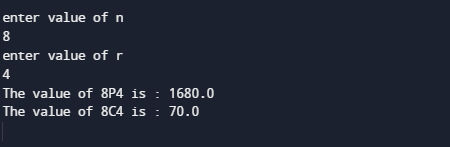In the above program, we have first declared and initialized a set variables required in the program.

• N= it will hold entered value of n
• R = it will hold entered value of r
• per = it will give value of permutation.
• com= it will give value of Combination.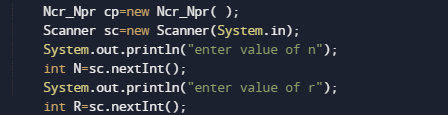First of all we declared and initialized set of required variables in the program.Then take a value from user at run time.Afterword we pass the given values to function named cp.permutation(n,r) it calls the permut() method and permut() method calls the fact() method and prints all the permutations of numbers.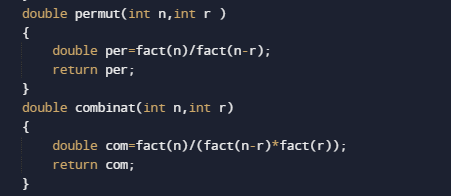cp.combinat(n,r) it will calls the combinat() method and combinat() method calls the fact()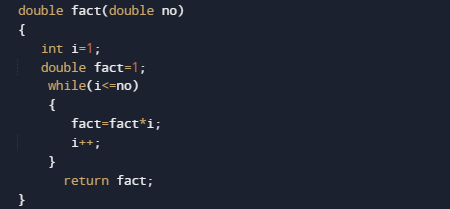method and prints all the Combinations.this process will keep on repeating until the condition if(n>=r) became false.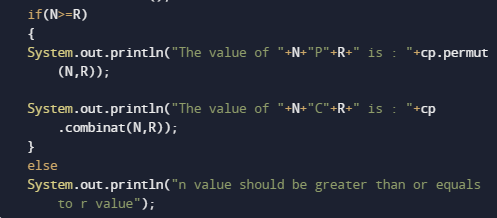And at last we will print all the value or Permutation and Combination.

In this tutorial we have learn about the Java Program to Find Ncr and Npr and its application with practical example. I hope you will like this tutorial.